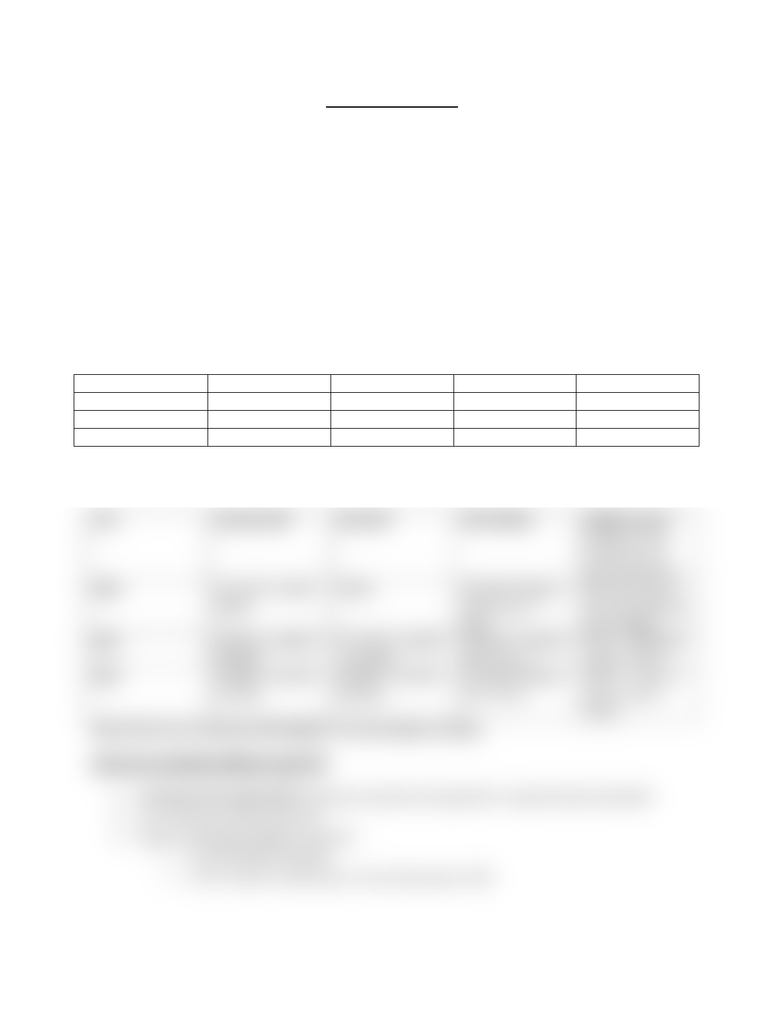Textbook Notes (280,000)
CA (160,000)
McMaster (10,000)
ECON (800)
ECON 1BB3 (300)
Chapter 5

ECON 1BB3 Chapter Notes - Chapter 5: Gdp Deflator

Department
Economics
Course Code
ECON 1BB3
Professor
Bridget O' Shaughnessy
Chapter
5

This preview shows half of the first page. to view the full 2 pages of the document.Richard Damra Thursday, January 24, 2013
Econ 1BB3 Chapter 5
How do I know if I’m better off than my parents?
GDP increases if prices increases or if output increases
Real GDP vs nominal GDP (base year prices vs current year prices)
The only way real GDP goes up over time is if we produce more stuff. Nominal goes up over
time if output or prices goes up.
GDP Deflator: a measure of the price level
GDP Deflator = (nominal GDP / real GDP) x 100
Example: Canada’s GDP is based on only two goods: skates and hockey pucks. Calculate GDP
with the data below.
Skates Hockey Pucks
Year
Price
Quantity
Price
Quantity
2010
\$50
100
\$3
500
2011
\$60
200
\$4
1000
2012
\$70
300
\$5
1200
Base year = 2010
Year
Nominal GDP
Real GDP
GDP deflator
Inflation rate(%
change in price
level from one
year to the next)
2010
50 x 100 + 3x500 =
\$6500
\$6500
100 (GDP deflator
of base year =
100)
N/A (we have no
idea what Inflation
was in 2009)
2011
60x200 + 4x1080 =
\$16 000
50 x 200 + 3x1000
= \$13 000
(16 000 / 13 000) x
100 = 123.1
((123 100)/100)
x 100 = 23.1%
2012
70x300 + 5x1200 =
\$27 000
50x300 + 3x1200 =
\$18 600
(27 000/ 18 600) x
100 = 145.2
((145.2 123.1) /
123.1) x 100 =
18.0%
Note: there are no units for GDP deflator, it is just simply a number
How do we calculate inflation using CPI?
Consumer Price Index (CPI): measures overall cost of goods for a typical urban household
It is a measure of the price level
Steps to calculating inflation using CPI
o 1. Fix the basket of goods
o 2. CPI = (cost in current year / cost in base year) x 100
You're Reading a Preview

Unlock to view full version